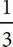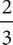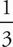# SAT Math Multiple Choice Question 63: Answer and Explanation

### Test Information

Question: 63

3. Syed took out a cash advance of d dollars from a financing company. The company deducts a fee ofof the original advanced amount along with a wire transfer fee of \$30.00. Which of the following represents the final advanced amount that Syed receives after all applied fees, in dollars?

• A.d - 30
• B.(d - 30)
• C.(d - 30)
• D.d - 30

D Whenever there are variables in the question, plug in. Be sure to plug in a number that is divisible by 3. Let d = 300.of the original amount of \$300 is \$100, and that is deducted by the company, leaving Syed with \$200. Now, subtract the wire transfer fee to get \$200 - \$30 = \$170, which is the target number. Plug in 300 for d in the answers to see which answer is equal to the target number of 170. In (A),(300) - 30 = 70. This is not the target number, so eliminate (A). Likewise in (B),(300 - 30) = 90, and in (C),(300 - 30) = 180. Neither of these is the target number, so eliminate (B) and (C). In (D),(300) - 30 = 170, which is the target number. The correct answer is (D).